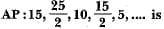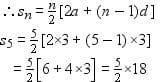RS Aggarwal Test: Arithmetic Progressions

# RS Aggarwal Test: Arithmetic Progressions

Test Description

## 10 Questions MCQ Test Mathematics (Maths) Class 10 | RS Aggarwal Test: Arithmetic Progressions

RS Aggarwal Test: Arithmetic Progressions for Class 10 2022 is part of Mathematics (Maths) Class 10 preparation. The RS Aggarwal Test: Arithmetic Progressions questions and answers have been prepared according to the Class 10 exam syllabus.The RS Aggarwal Test: Arithmetic Progressions MCQs are made for Class 10 2022 Exam. Find important definitions, questions, notes, meanings, examples, exercises, MCQs and online tests for RS Aggarwal Test: Arithmetic Progressions below.
Solutions of RS Aggarwal Test: Arithmetic Progressions questions in English are available as part of our Mathematics (Maths) Class 10 for Class 10 & RS Aggarwal Test: Arithmetic Progressions solutions in Hindi for Mathematics (Maths) Class 10 course. Download more important topics, notes, lectures and mock test series for Class 10 Exam by signing up for free. Attempt RS Aggarwal Test: Arithmetic Progressions | 10 questions in 10 minutes | Mock test for Class 10 preparation | Free important questions MCQ to study Mathematics (Maths) Class 10 for Class 10 Exam | Download free PDF with solutions
 1 Crore+ students have signed up on EduRev. Have you?
RS Aggarwal Test: Arithmetic Progressions - Question 1

### The 11th and 13th terms of an AP are 35 and 41 respectively, its common difference is

Detailed Solution for RS Aggarwal Test: Arithmetic Progressions - Question 1

11th term is 35 so a+10d = 35 => equation 1

13th term is 41 so a+12d = 41 => equation 2

solve both equations by elimination method

we get common difference as 3

RS Aggarwal Test: Arithmetic Progressions - Question 2

### An AP consists of 31 terms. If its 16th term is m, then sum of all the terms of this AP is

Detailed Solution for RS Aggarwal Test: Arithmetic Progressions - Question 2

S31 = 31/2(2a + 30d)
a16 = a + 15d = m
⇒ S31 = (31/2) x 2(a + 15d) ⇒ S31 = 31 m

RS Aggarwal Test: Arithmetic Progressions - Question 3

### Which term of the AP: 18, 23, 28, 33,... is 98?

Detailed Solution for RS Aggarwal Test: Arithmetic Progressions - Question 3

98=a+(n-1)d

98=18+(n-1)5

80=(n-1)5

16=n-1

17=n

this explains that 98 is 17th term of the AP

RS Aggarwal Test: Arithmetic Progressions - Question 4

The 16th term of theDetailed Solution for RS Aggarwal Test: Arithmetic Progressions - Question 4

A= 15
d = a2 - a1
d = 25 / 2 - 15
d = ( 30 - 25 / 2 )
d = - 5/ 2
16th term of an AP = a + 15 d
15 +( 15 × ( -5 / 2 ))
15 + (-75 / 2 )
= - 45 / 2

RS Aggarwal Test: Arithmetic Progressions - Question 5

The list of numbers -10, -6, -2, 2,... is

Detailed Solution for RS Aggarwal Test: Arithmetic Progressions - Question 5

a1=-10
a2=-6
so the common difference
d=(-6-(-10)) = 4

RS Aggarwal Test: Arithmetic Progressions - Question 6

The sum of first 16 terms of the AP : 10, 6, 2,... is

Detailed Solution for RS Aggarwal Test: Arithmetic Progressions - Question 6

The formula for the sum of first n terms of a AP is n/2(2a+(n-1)d)
here n=16 a=10 d=6-10=2-6=-4
so sum of first 16 terms=16/2(2·10+(16-1)·-4)
=8(20+15·-4)
=8(20-60)
=8(-40)
=-320

RS Aggarwal Test: Arithmetic Progressions - Question 7

The sum of first five multiples of 3 is

Detailed Solution for RS Aggarwal Test: Arithmetic Progressions - Question 7

First five multiple of 3 are:

3, 6, 9, 12, 15

Here first term, a = 3

common difference, d = 6 – 3 = 3

Number of term, n = 5⇒ s5 = 9 × 5 = 45

RS Aggarwal Test: Arithmetic Progressions - Question 8

Two APs have same common difference. The first term of one of these is -1 and that of the other is - 8. Then the difference between their 4th term is

Detailed Solution for RS Aggarwal Test: Arithmetic Progressions - Question 8

Correct Answer :- c

Explanation : ad1 = -1

4th term of ad1 is

a4 = a + 3d

a4 = -1 + 3d.....(1)

a4 = -8 + 3d.......(2)

Subtracting (2) from (1), we get

-1 + 3d -(-8 + 3d)

=> 7

RS Aggarwal Test: Arithmetic Progressions - Question 9

The 21st term of the AP whose first two terms are -3 and 4, is

Detailed Solution for RS Aggarwal Test: Arithmetic Progressions - Question 9

a1=-3,
a2=4,
d=4-(-3)=7,
a21=?,
a21=a+(n-1)d
=-3+(21-1)7
= -3+(20)(7)
= -3+140
=137

RS Aggarwal Test: Arithmetic Progressions - Question 10

If 18, a, b, -3 are in AP, then a + b is equal to

Detailed Solution for RS Aggarwal Test: Arithmetic Progressions - Question 10

We know

AM  is ( a + b)/2

(a+b) = [ {18+(-3) }/2] X 2 = 15

## Mathematics (Maths) Class 10

53 videos|369 docs|138 tests
 Use Code STAYHOME200 and get INR 200 additional OFF Use Coupon Code
Information about RS Aggarwal Test: Arithmetic Progressions Page
In this test you can find the Exam questions for RS Aggarwal Test: Arithmetic Progressions solved & explained in the simplest way possible. Besides giving Questions and answers for RS Aggarwal Test: Arithmetic Progressions, EduRev gives you an ample number of Online tests for practice

## Mathematics (Maths) Class 10

53 videos|369 docs|138 tests